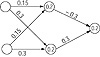# 35.C++ Neural Networks and Fuzzy Logic by Valluru B. Rao M&T Books, IDG Books Worldwide, Inc. ISBN: 1558515526   Pub Date: 06/01/95

#### A Second Look at the XOR Function: Multilayer Perceptron

By introducing a set of cascaded Perceptrons, you have a Perceptron network, with an input layer, middle or hidden layer, and an output layer. You will see that the multilayer Perceptron can evaluate the XOR function as well as other logic functions (AND, OR, MAJORITY, etc.). The absence of the separability that we talked about earlier is overcome by having a second stage, so to speak, of connection weights.

You need two neurons in the input layer and one in the output layer. Let us put a hidden layer with two neurons. Let w11, w12, w21, and w22, be the weights on connections from the input neurons to the hidden layer neurons. Let v1, v2 , be the weights on the connections from the hidden layer neurons to the outout neuron.

We will select the w’s (weights) and the threshold values θ1 , and θ2 at the hidden layer neurons, so that the input (0, 0) generates the output vector (0, 0), and the input vector (1, 1) generates (1, 1), while the inputs (1, 0) and (0, 1) generate (0, 1) as the hidden layer output. The inputs to the output layer neurons would be from the set {(0, 0), (1, 1), (0, 1)}. These three vectors are separable, with (0, 0), and (1, 1) on one side of the separating line, while (0, 1) is on the other side.

We will select the √s (weights) and τ, the threshold value at the output neuron, so as to make the inputs (0, 0) and (1, 1) cause an output of 0 for the network, and an output of 1 is caused by the input (0, 1). The network layout within the labels of weights and threshold values inside the nodes representing hidden layer and output neurons is shown in Figure 5.1a. Table 5.2 gives the results of operation of this network.Figure 5.1a  Example network.

Table 5.2 Results for the Perceptron with One Hidden Layer.

Input Hidden Layer Activations Hidden Layer Outputs Output Neuron activaton Output of network
(0, 0) (0, 0) (0, 0) 0 0
(1, 1) (0.3, 0.6) (1, 1) 0 0
(0, 1) (0.15, 0.3) (0, 1) 0.3 1
(1, 0) (0.15, 0.3) (0, 1) 0.3 1

It is clear from Table 5.2, that the above perceptron with a hidden layer does compute the XOR function successfully.

Note:  The activation should exceed the threshold value for a neuron to fire. Where the output of a neuron is shown to be 0, it is because the internal activation of that neuron fell short of its threshold value.

#### Example of the Cube Revisited

Let us return to the example of the cube with vertices at the origin O, and the points labeled A, B, C, D, E, F, and G. Suppose the set of vertices O, A, F, and G give a value of 1 for the function to be evaluated, and the other vertices give a –1. The two sets are not linearly separable as mentioned before. A simple Perceptron cannot evaluate this function.

Can the addition of another layer of neurons help? The answer is yes. What would be the role of this additional layer? The answer is that it will do the final processing for the problem after the previous layer has done some preprocessing. This can do two separations in the sense that the set of eight vertices can be separated—or partitioned—into three separable subsets. If this partitioning can also help collect within each subset, like vertices, meaning those that map onto the same value for the function, the network will succeed in its task of evaluating the function when the aggregation and thresholding is done at the output neuron.

#### Strategy

So the strategy is first to consider the set of vertices that give a value of +1 for the function and determine the minimum number of subsets that can be identified to be each separable from the rest of the vertices. It is evident that since the vertices O and A lie on one edge of the cube, they can form one subset that is separable. The other two vertices, viz., F and one for G, which correspond to the value +1 for the function, can form a second subset that is separable, too. We need not bother with the last four vertices from the point of view of further partitioning that subset. It is clear that one new layer of three neurons, one of which fires for the inputs corresponding to the vertices O and A, one for F, and G, and the third for the rest, will then facilitate the correct evaluation of the function at the output neuron.C++ Neural Networks and Fuzzy Logic
ISBN: 1558515526
EAN: 2147483647
Year: 1995
Pages: 139

Similar book on Amazon